×
Get Full Access to Chemistry: A Molecular Approach - 3 Edition - Chapter 1 - Problem 88ae
Get Full Access to Chemistry: A Molecular Approach - 3 Edition - Chapter 1 - Problem 88ae

×

# In 2009, a team from Northwestern University and WesternISBN: 9780321809247 1

## Solution for problem 88AE Chapter 1

Chemistry: A Molecular Approach | 3rd Edition

• Textbook Solutions
• 2901 Step-by-step solutions solved by professors and subject experts
• Get 24/7 help from StudySoup virtual teaching assistantsChemistry: A Molecular Approach | 3rd Edition

4 5 1 255 Reviews
12
3
Problem 88AE

In 2009, a team from Northwestern University and Western Washington University reported the preparation of a new ‘spongy” material composed of nickel, molybdenum, and sulfur that excels at removing mercury from water. The density of this new material is 0.20 g/cm3, and its surface area is 1242 m2 per gram of material, (a) Calculate the volume of a 10.0-mg sample of this material, (b) Calculate the surface area for a 10.0-mg sample of this material, (c) A 10.0-mL sample of contaminated water had 7.748 mg of mercury in it. After treatment with 10.0 mg of the new spongy material, 0.001 mg of mercury remained in the contaminated water. What percentage of the mercury was removed from the water? (d) What is the final mass of the spongy material after the exposure to mercury?

Step-by-Step Solution:

Step 1 of 4

(a)

From the given,

Mass of material = 10.0 g

Volume of the material =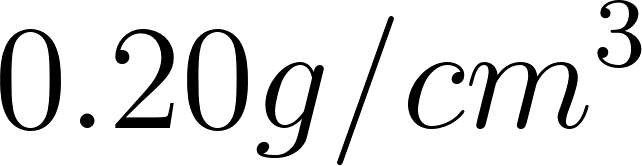Let’s convert the density grams into milligrams.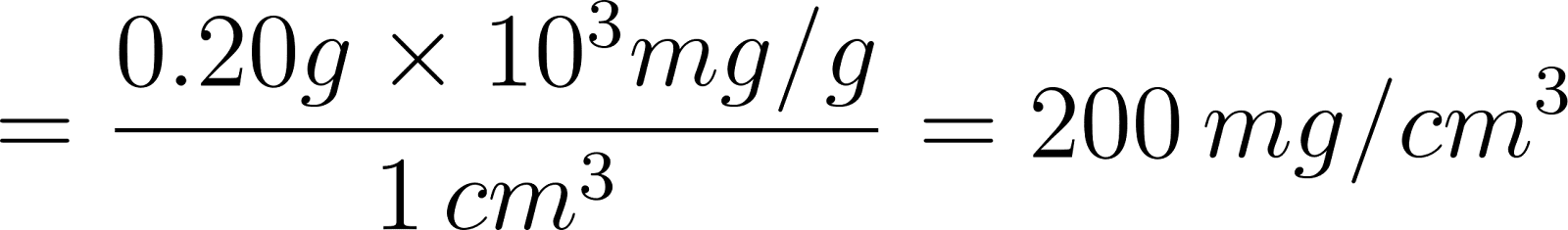Density can be calculated by the following formula;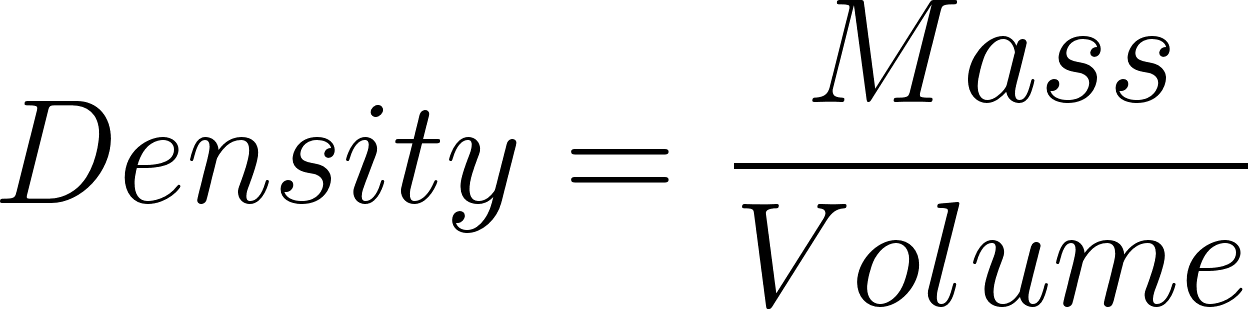..................(1)

Rearrange the formula (1)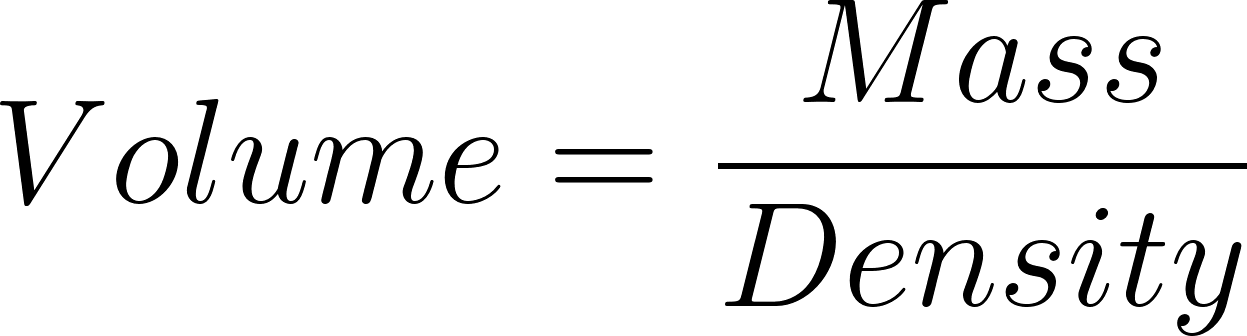......................(2)

Substitute the values in the formula (2)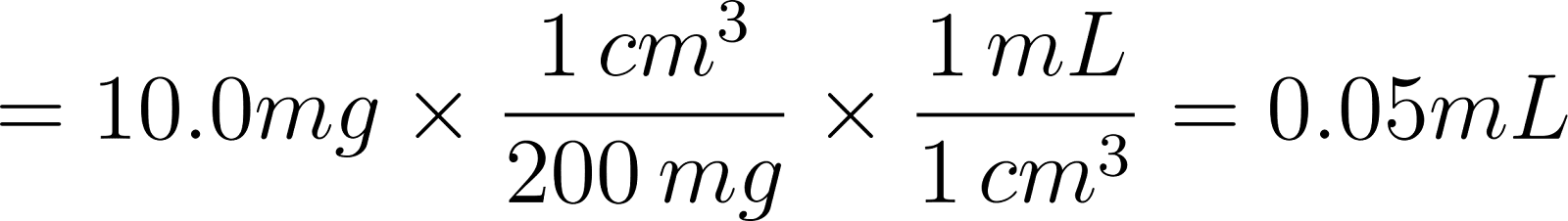Therefore, the volume of the new material is 0.050 mL .

__________________________________________________________________________

Step 2 of 4

Step 3 of 4

##### ISBN: 9780321809247

Since the solution to 88AE from 1 chapter was answered, more than 1083 students have viewed the full step-by-step answer. This textbook survival guide was created for the textbook: Chemistry: A Molecular Approach, edition: 3. The answer to “?In 2009, a team from Northwestern University and Western Washington University reported the preparation of a new ‘spongy” material composed of nickel, molybdenum, and sulfur that excels at removing mercury from water. The density of this new material is 0.20 g/cm3, and its surface area is 1242 m2 per gram of material, (a) Calculate the volume of a 10.0-mg sample of this material, (b) Calculate the surface area for a 10.0-mg sample of this material, (c) A 10.0-mL sample of contaminated water had 7.748 mg of mercury in it. After treatment with 10.0 mg of the new spongy material, 0.001 mg of mercury remained in the contaminated water. What percentage of the mercury was removed from the water? (d) What is the final mass of the spongy material after the exposure to mercury?” is broken down into a number of easy to follow steps, and 133 words. Chemistry: A Molecular Approach was written by and is associated to the ISBN: 9780321809247. This full solution covers the following key subjects: material, mercury, Water, new, sample. This expansive textbook survival guide covers 82 chapters, and 9454 solutions. The full step-by-step solution to problem: 88AE from chapter: 1 was answered by , our top Chemistry solution expert on 02/22/17, 04:35PM.

## Discover and learn what students are asking

Calculus: Early Transcendental Functions : Differentiation and Integration of Vector-Valued Functions
?Differentiation of Vector-Valued Functions In Exercises 1-6, find r’(t), $$\mathrm{r}\left(t_{0}\right)$$, and $$\mathrm{r’}\left(t_{0}\right)$$ for t

Statistics: Informed Decisions Using Data : Properties of the Normal Distribution
?In Problems 25–28, the graph of a normal curve is given. Use the graph to identify the values of ? and ?.

Unlock Textbook Solution